## C++初学者学习笔记

news/2023/6/7 23:38:49

# 面向对象的程序设计

## C++ 简介

C++ 是一种静态类型的、编译式的、通用的、大小写敏感的、不规则的编程语言，支持过程化编程、面向对象编程和泛型编程。

C++ 被认为是一种中级语言，它综合了高级语言和低级语言的特点。

C++ 是由 Bjarne Stroustrup 于 1979 年在新泽西州美利山贝尔实验室开始设计开发的。C++ 进一步扩充和完善了 C 语言，最初命名为带类的C，后来在 1983 年更名为 C++。

C++ 是 C 的一个超集，事实上，任何合法的 C 程序都是合法的 C++ 程序。

## 面向对象程序设计

C++ 完全支持面向对象的程序设计，包括面向对象开发的四大特性：

## C++ 的使用

C++ 通常用于编写设备驱动程序和其他要求实时性的直接操作硬件的软件。

## C++语言基础

C++ 程序可以定义为对象的集合，这些对象通过调用彼此的方法进行交互。现在让我们简要地看一下什么是类、对象，方法、即时变量。

:类可以定义为描述对象行为/状态的模板/蓝图。

### 在 C++ 中 main 函数前面为什么要加上数据类型，比如： int void ?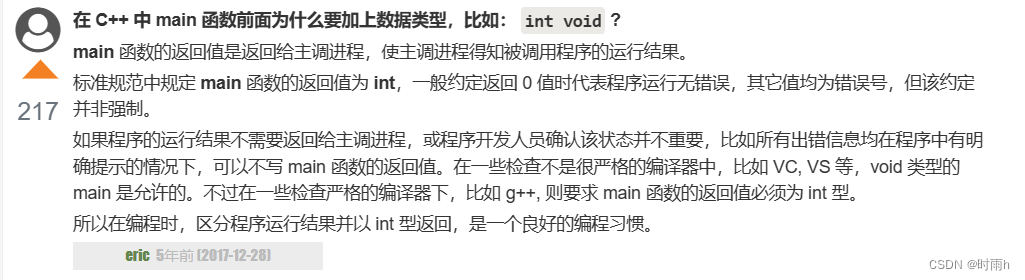## C++ 注释

C++ 注释一般有两种：

// - 一般用于单行注释。

/* … */ - 一般用于多行注释。

## C++数据类型

C++ 数据类型

``````#include<iostream>
#include <limits>using namespace std;  int main()
{  cout << "type: \t\t" << "************size**************"<< endl;  cout << "bool: \t\t" << "所占字节数：" << sizeof(bool);  cout << "\t最大值：" << (numeric_limits<bool>::max)();  cout << "\t\t最小值：" << (numeric_limits<bool>::min)() << endl;  cout << "char: \t\t" << "所占字节数：" << sizeof(char);  cout << "\t最大值：" << (numeric_limits<char>::max)();  cout << "\t\t最小值：" << (numeric_limits<char>::min)() << endl;  cout << "signed char: \t" << "所占字节数：" << sizeof(signed char);  cout << "\t最大值：" << (numeric_limits<signed char>::max)();  cout << "\t\t最小值：" << (numeric_limits<signed char>::min)() << endl;  cout << "unsigned char: \t" << "所占字节数：" << sizeof(unsigned char);  cout << "\t最大值：" << (numeric_limits<unsigned char>::max)();  cout << "\t\t最小值：" << (numeric_limits<unsigned char>::min)() << endl;  cout << "wchar_t: \t" << "所占字节数：" << sizeof(wchar_t);  cout << "\t最大值：" << (numeric_limits<wchar_t>::max)();  cout << "\t\t最小值：" << (numeric_limits<wchar_t>::min)() << endl;  cout << "short: \t\t" << "所占字节数：" << sizeof(short);  cout << "\t最大值：" << (numeric_limits<short>::max)();  cout << "\t\t最小值：" << (numeric_limits<short>::min)() << endl;  cout << "int: \t\t" << "所占字节数：" << sizeof(int);  cout << "\t最大值：" << (numeric_limits<int>::max)();  cout << "\t最小值：" << (numeric_limits<int>::min)() << endl;  cout << "unsigned: \t" << "所占字节数：" << sizeof(unsigned);  cout << "\t最大值：" << (numeric_limits<unsigned>::max)();  cout << "\t最小值：" << (numeric_limits<unsigned>::min)() << endl;  cout << "long: \t\t" << "所占字节数：" << sizeof(long);  cout << "\t最大值：" << (numeric_limits<long>::max)();  cout << "\t最小值：" << (numeric_limits<long>::min)() << endl;  cout << "unsigned long: \t" << "所占字节数：" << sizeof(unsigned long);  cout << "\t最大值：" << (numeric_limits<unsigned long>::max)();  cout << "\t最小值：" << (numeric_limits<unsigned long>::min)() << endl;  cout << "double: \t" << "所占字节数：" << sizeof(double);  cout << "\t最大值：" << (numeric_limits<double>::max)();  cout << "\t最小值：" << (numeric_limits<double>::min)() << endl;  cout << "long double: \t" << "所占字节数：" << sizeof(long double);  cout << "\t最大值：" << (numeric_limits<long double>::max)();  cout << "\t最小值：" << (numeric_limits<long double>::min)() << endl;  cout << "float: \t\t" << "所占字节数：" << sizeof(float);  cout << "\t最大值：" << (numeric_limits<float>::max)();  cout << "\t最小值：" << (numeric_limits<float>::min)() << endl;  cout << "size_t: \t" << "所占字节数：" << sizeof(size_t);  cout << "\t最大值：" << (numeric_limits<size_t>::max)();  cout << "\t最小值：" << (numeric_limits<size_t>::min)() << endl;  cout << "string: \t" << "所占字节数：" << sizeof(string) << endl;  // << "\t最大值：" << (numeric_limits<string>::max)() << "\t最小值：" << (numeric_limits<string>::min)() << endl;  cout << "type: \t\t" << "************size**************"<< endl;  return 0;
}
``````

### typedef 声明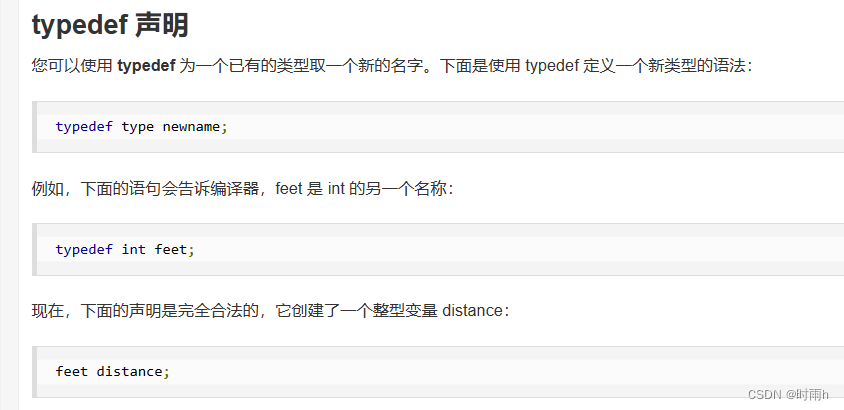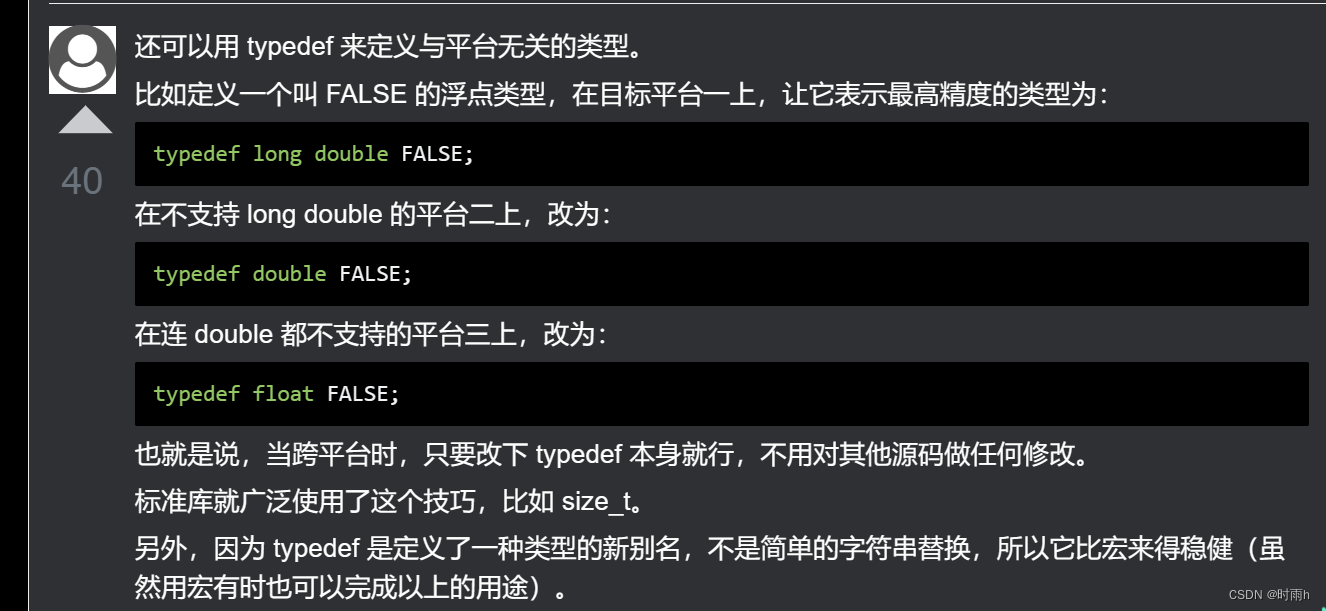### 枚举类型

``````enum 枚举名{ 标识符[=整型常数], 标识符[=整型常数],
... 标识符[=整型常数]
} 枚举变量;
``````

enum color { red, green, blue } c;
c = blue;

enum color { red, green=5, blue };

### typedef 与 #define 的区别

1. 执行时间不同

#define 则是宏定义，发生在预处理阶段，也就是编译之前，它只进行简单而机械的字符串替换，而不进行任何检查。

【例1.1】typedef 会做相应的类型检查：

``````typedef unsigned int UINT;void func()
{UINT value = "abc"; // error C2440: 'initializing' : cannot convert from 'const char ' to 'UINT'cout << value << endl;
}
【例1.2】#define不做类型检查：// #define用法例子：
#define f(x) x*x
int main()
{int a=6, b=2, c;c=f(a) / f(b);printf("%d\n", c);return 0;
}
``````

2、功能有差异

typedef 用来定义类型的别名，定义与平台无关的数据类型，与 struct 的结合使用等。

#define 不只是可以为类型取别名，还可以定义常量、变量、编译开关等。

3、作用域不同

#define 没有作用域的限制，只要是之前预定义过的宏，在以后的程序中都可以使用。

【例3.1】没有作用域的限制，只要是之前预定义过就可以

``````void func1()
{#define HW "HelloWorld";
}void func2()
{string str = HW;cout << str << endl;
}
【例3.2】而typedef有自己的作用域void func1()
{typedef unsigned int UINT;
}void func2()
{UINT uValue = 5;//error C2065: 'UINT' : undeclared identifier
}
``````

【例3.3】

``````class A
{typedef unsigned int UINT;UINT valueA;A() : valueA(0){}
};class B
{UINT valueB;//error C2146: syntax error : missing ';' before identifier 'valueB'//error C4430: missing type specifier - int assumed. Note: C++ does not support default-int
};
``````

``````class A
{typedef unsigned int UINT;UINT valueA;A() : valueA(0){}
};void func3()
{A::UINT i = 1;// error C2248: 'A::UINT' : cannot access private typedef declared in class 'A'
}
``````

【例3.5】：

``````class A
{
public:typedef unsigned int UINT;UINT valueA;A() : valueA(0){}
};void func3()
{A::UINT i = 1;cout << i << endl;
}
``````

4、对指针的操作

typedef int * pint;
#define PINT int *

int i1 = 1, i2 = 2;

const pint p1 = &i1; //p不可更改，p指向的内容可以更改，相当于 int * const p;
const PINT p2 = &i2; //p可以更改，p指向的内容不能更改，相当于 const int *p；或 int const *p；

pint s1, s2; //s1和s2都是int型指针
PINT s3, s4; //相当于int * s3，s4；只有一个是指针。

``````void TestPointer()
{cout << "p1:" << p1 << "  *p1:" << *p1 << endl;//p1 = &i2; //error C3892: 'p1' : you cannot assign to a variable that is const*p1 = 5;cout << "p1:" << p1 << "  *p1:" << *p1 << endl;cout << "p2:" << p2 << "  *p2:" << *p2 << endl;//*p2 = 10; //error C3892: 'p2' : you cannot assign to a variable that is constp2 = &i1;cout << "p2:" << p2 << "  *p2:" << *p2 << endl;
}
``````

p1:00EFD094 *p1:1
p1:00EFD094 *p1:5
p2:00EFD098 *p2:2
p2:00EFD094 *p2:5

C++ 变量类型

## C++ 变量作用域

``````#include <iostream>
using namespace std;// 全局变量声明
int g;int main ()
{// 局部变量声明int a, b;// 实际初始化a = 10;b = 20;g = a + b;cout << g;return 0;
}
``````

## C++ 常量

### 整数常量

212 // 合法的
215u // 合法的
0xFeeL // 合法的
078 // 非法的：8 不是八进制的数字
032UU // 非法的：不能重复后缀

85 // 十进制
0213 // 八进制
0x4b // 十六进制
30 // 整数
30u // 无符号整数
30l // 长整数
30ul // 无符号长整数

### 浮点常量

3.14159 // 合法的
314159E-5L // 合法的
510E // 非法的：不完整的指数
210f // 非法的：没有小数或指数
.e55 // 非法的：缺少整数或分数

### 字符常量

\ \ 字符
’ ’ 字符
" " 字符
? ? 字符
\a 警报铃声
\b 退格键
\f 换页符
\n 换行符
\r 回车
\t 水平制表符
\v 垂直制表符
\ooo 一到三位的八进制数
\xhh . . . 一个或多个数字的十六进制数

### 定义常量

Bjarne在他的The C++ Programming Language里面给出过一个助记的方法：把一个声明从右向左读。

char * const cp; ( * 读成 pointer to )
cp is a const pointer to char

const char * p;
p is a pointer to const char;

char const * p;

const char*, char const*, char*const 的区别

## C++ 修饰符类型

C++ 允许在 char、int 和 double 数据类型前放置修饰符。修饰符用于改变基本类型的含义，所以它更能满足各种情境的需求。

signed
unsigned
long
short

C++ 允许使用速记符号来声明无符号短整数或无符号长整数。您可以不写 int，只写单词 unsigned、short 或 long，int 是隐含的。例如，下面的两个语句都声明了无符号整型变量。

unsigned x;
unsigned int y;

### C++ 中的类型限定符

const const 类型的对象在程序执行期间不能被修改改变。
volatile 修饰符 volatile 告诉编译器不需要优化volatile声明的变量，让程序可以直接从内存中读取变量。对于一般的变量编译器会对变量进行优化，将内存中的变量值放在寄存器中以加快读写效率。
restrict 由 restrict 修饰的指针是唯一一种访问它所指向的对象的方式。只有 C99 增加了新的类型限定符 restrict。

## C++ 存储类

### auto 存储类

C++98标准中auto关键字用于自动变量的声明，但由于使用极少且多余，在 C++17 中已删除这一用法。

auto f=3.14; //double
auto s(“hello”); //const char*
auto z = new auto(9); // int*
auto x1 = 5, x2 = 5.0, x3=‘r’;//错误，必须是初始化为同一类型

### register 存储类

register 存储类用于定义存储在寄存器中而不是 RAM 中的局部变量。这意味着变量的最大尺寸等于寄存器的大小（通常是一个词），且不能对它应用一元的 ‘&’ 运算符（因为它没有内存位置）。

{
register int miles;
}

### static 存储类

static 存储类指示编译器在程序的生命周期内保持局部变量的存在，而不需要在每次它进入和离开作用域时进行创建和销毁。因此，使用 static 修饰局部变量可以在函数调用之间保持局部变量的值。

static 修饰符也可以应用于全局变量。当 static 修饰全局变量时，会使变量的作用域限制在声明它的文件内。

#include

// 函数声明
void func(void);

static int count = 10; /* 全局变量 */

int main()
{
while(count–)
{
func();
}
return 0;
}
// 函数定义
void func( void )
{
static int i = 5; // 局部静态变量
i++;
std::cout << "变量 i 为 " << i ;
std::cout << " , 变量 count 为 " << count << std::endl;
}

### extern 存储类

extern 存储类用于提供一个全局变量的引用，全局变量对所有的程序文件都是可见的。当您使用 ‘extern’ 时，对于无法初始化的变量，会把变量名指向一个之前定义过的存储位置。

extern 修饰符通常用于当有两个或多个文件共享相同的全局变量或函数的时候，如下所示：

#include

int count ;
extern void write_extern();

int main()
{
count = 5;
write_extern();
}

#include

extern int count;

void write_extern(void)
{
std::cout << "Count is " << count << std::endl;
}

\$ g++ main.cpp support.cpp -o write

\$ ./write
Count is 5

### mutable 存储类

mutable 说明符仅适用于类的对象，这将在本教程的最后进行讲解。它允许对象的成员替代常量。也就是说，mutable 成员可以通过 const 成员函数修改。

thread_local 说明符可以与 static 或 extern 合并。

class X
{
static thread_local std::string s; // 类的static成员变量
};
static thread_local std::string X:😒; // X:😒 是需要定义的

void foo()
{
}

## C++ 运算符

### 算术运算符

``````+	把两个操作数相加	A + B 将得到 30
-	从第一个操作数中减去第二个操作数	A - B 将得到 -10
*	把两个操作数相乘	A * B 将得到 200
/	分子除以分母	B / A 将得到 2
%	取模运算符，整除后的余数	B % A 将得到 0
++	自增运算符，整数值增加 1	A++ 将得到 11
--	自减运算符，整数值减少 1	A-- 将得到 9
``````

``````#include <iostream>
using namespace std;int main()
{int a = 21;int b = 10;int c;c = a + b;cout << "Line 1 - c 的值是 " << c << endl ;c = a - b;cout << "Line 2 - c 的值是 " << c << endl ;c = a * b;cout << "Line 3 - c 的值是 " << c << endl ;c = a / b;cout << "Line 4 - c 的值是 " << c << endl ;c = a % b;cout << "Line 5 - c 的值是 " << c << endl ;int d = 10;   //  测试自增、自减c = d++;cout << "Line 6 - c 的值是 " << c << endl ;d = 10;    // 重新赋值c = d--;cout << "Line 7 - c 的值是 " << c << endl ;return 0;
}
``````

Line 1 - c 的值是 31
Line 2 - c 的值是 11
Line 3 - c 的值是 210
Line 4 - c 的值是 2
Line 5 - c 的值是 1
Line 6 - c 的值是 10
Line 7 - c 的值是 10

### 关系运算符

``````==	检查两个操作数的值是否相等，如果相等则条件为真。	(A == B) 不为真。
!=	检查两个操作数的值是否相等，如果不相等则条件为真。	(A != B) 为真。
>	检查左操作数的值是否大于右操作数的值，如果是则条件为真。	(A > B) 不为真。
<	检查左操作数的值是否小于右操作数的值，如果是则条件为真。	(A < B) 为真。
>=	检查左操作数的值是否大于或等于右操作数的值，如果是则条件为真。	(A >= B) 不为真。
<=	检查左操作数的值是否小于或等于右操作数的值，如果是则条件为真。	(A <= B) 为真。
``````

``````#include <iostream>
using namespace std;int main()
{int a = 21;int b = 10;int c ;if( a == b ){cout << "Line 1 - a 等于 b" << endl ;}else{cout << "Line 1 - a 不等于 b" << endl ;}if ( a < b ){cout << "Line 2 - a 小于 b" << endl ;}else{cout << "Line 2 - a 不小于 b" << endl ;}if ( a > b ){cout << "Line 3 - a 大于 b" << endl ;}else{cout << "Line 3 - a 不大于 b" << endl ;}/* 改变 a 和 b 的值 */a = 5;b = 20;if ( a <= b ){cout << "Line 4 - a 小于或等于 b" << endl ;}if ( b >= a ){cout << "Line 5 - b 大于或等于 a" << endl ;}return 0;
}
``````

Line 1 - a 不等于 b
Line 2 - a 不小于 b
Line 3 - a 大于 b
Line 4 - a 小于或等于 b
Line 5 - b 大于或等于 a

### 逻辑运算符

&& 称为逻辑与运算符。如果两个操作数都 true，则条件为 true。 (A && B) 为 false。
|| 称为逻辑或运算符。如果两个操作数中有任意一个 true，则条件为 true。 (A || B) 为 true。
! 称为逻辑非运算符。用来逆转操作数的逻辑状态，如果条件为 true 则逻辑非运算符将使其为 false。 !(A && B) 为 true。

``````实例
#include <iostream>
using namespace std;int main()
{int a = 5;int b = 20;int c ;if ( a && b ){cout << "Line 1 - 条件为真"<< endl ;}if ( a || b ){cout << "Line 2 - 条件为真"<< endl ;}/* 改变 a 和 b 的值 */a = 0;b = 10;if ( a && b ){cout << "Line 3 - 条件为真"<< endl ;}else{cout << "Line 4 - 条件不为真"<< endl ;}if ( !(a && b) ){cout << "Line 5 - 条件为真"<< endl ;}return 0;
}
``````

Line 1 - 条件为真
Line 2 - 条件为真
Line 4 - 条件不为真
Line 5 - 条件为真

### 位运算符

p q p & q p | q p ^ q
0 0 0 0 0
0 1 0 1 1
1 1 1 1 0
1 0 0 1 1

A = 0011 1100

B = 0000 1101

A&B = 0000 1100

A|B = 0011 1101

A^B = 0011 0001

~A = 1100 0011

``````运算符	描述	实例
&

0&1=0;
1&0=0;
1&1=1;	(A & B) 将得到 12，即为 0000 1100
|

0|1=1;
1|0=1;
1|1=1;	(A | B) 将得到 61，即为 0011 1101
^

0^1=1;
1^0=1;
1^1=0;	(A ^ B) 将得到 49，即为 0011 0001
~

~0=-1;	(~A ) 将得到 -61，即为 1100 0011，一个有符号二进制数的补码形式。
<<	二进制左移运算符。将一个运算对象的各二进制位全部左移若干位（左边的二进制位丢弃，右边补0）。	A << 2 将得到 240，即为 1111 0000
``````

``````#include <iostream>
using namespace std;int main()
{unsigned int a = 60;      // 60 = 0011 1100  unsigned int b = 13;      // 13 = 0000 1101int c = 0;           c = a & b;             // 12 = 0000 1100cout << "Line 1 - c 的值是 " << c << endl ;c = a | b;             // 61 = 0011 1101cout << "Line 2 - c 的值是 " << c << endl ;c = a ^ b;             // 49 = 0011 0001cout << "Line 3 - c 的值是 " << c << endl ;c = ~a;                // -61 = 1100 0011cout << "Line 4 - c 的值是 " << c << endl ;c = a << 2;            // 240 = 1111 0000cout << "Line 5 - c 的值是 " << c << endl ;c = a >> 2;            // 15 = 0000 1111cout << "Line 6 - c 的值是 " << c << endl ;return 0;
}
``````

Line 1 - c 的值是 12
Line 2 - c 的值是 61
Line 3 - c 的值是 49
Line 4 - c 的值是 -61
Line 5 - c 的值是 240
Line 6 - c 的值是 15

### 赋值运算符

``````=	简单的赋值运算符，把右边操作数的值赋给左边操作数	C = A + B 将把 A + B 的值赋给 C
+=	加且赋值运算符，把右边操作数加上左边操作数的结果赋值给左边操作数	C += A 相当于 C = C + A
-=	减且赋值运算符，把左边操作数减去右边操作数的结果赋值给左边操作数	C -= A 相当于 C = C - A
*=	乘且赋值运算符，把右边操作数乘以左边操作数的结果赋值给左边操作数	C *= A 相当于 C = C * A
/=	除且赋值运算符，把左边操作数除以右边操作数的结果赋值给左边操作数	C /= A 相当于 C = C / A
%=	求模且赋值运算符，求两个操作数的模赋值给左边操作数	C %= A 相当于 C = C % A
<<=	左移且赋值运算符	C <<= 2 等同于 C = C << 2
>>=	右移且赋值运算符	C >>= 2 等同于 C = C >> 2
&=	按位与且赋值运算符	C &= 2 等同于 C = C & 2
^=	按位异或且赋值运算符	C ^= 2 等同于 C = C ^ 2
|=	按位或且赋值运算符	C |= 2 等同于 C = C | 2
``````

``````
```cpp
#include <iostream>
using namespace std;int main()
{int a = 21;int c ;c =  a;cout << "Line 1 - =  运算符实例，c 的值 = : " <<c<< endl ;c +=  a;cout << "Line 2 - += 运算符实例，c 的值 = : " <<c<< endl ;c -=  a;cout << "Line 3 - -= 运算符实例，c 的值 = : " <<c<< endl ;c *=  a;cout << "Line 4 - *= 运算符实例，c 的值 = : " <<c<< endl ;c /=  a;cout << "Line 5 - /= 运算符实例，c 的值 = : " <<c<< endl ;c  = 200;c %=  a;cout << "Line 6 - %= 运算符实例，c 的值 = : " <<c<< endl ;c <<=  2;cout << "Line 7 - <<= 运算符实例，c 的值 = : " <<c<< endl ;c >>=  2;cout << "Line 8 - >>= 运算符实例，c 的值 = : " <<c<< endl ;c &=  2;cout << "Line 9 - &= 运算符实例，c 的值 = : " <<c<< endl ;c ^=  2;cout << "Line 10 - ^= 运算符实例，c 的值 = : " <<c<< endl ;c |=  2;cout << "Line 11 - |= 运算符实例，c 的值 = : " <<c<< endl ;return 0;
}
``````

Line 1 - = 运算符实例，c 的值 = 21
Line 2 - += 运算符实例，c 的值 = 42
Line 3 - -= 运算符实例，c 的值 = 21
Line 4 - *= 运算符实例，c 的值 = 441
Line 5 - /= 运算符实例，c 的值 = 21
Line 6 - %= 运算符实例，c 的值 = 11
Line 7 - <<= 运算符实例，c 的值 = 44
Line 8 - >>= 运算符实例，c 的值 = 11
Line 9 - &= 运算符实例，c 的值 = 2
Line 10 - ^= 运算符实例，c 的值 = 0
Line 11 - |= 运算符实例，c 的值 = 2

### 杂项运算符

``````sizeof	sizeof 运算符返回变量的大小。例如，sizeof(a) 将返回 4，其中 a 是整数。
Condition ? X : Y	条件运算符。如果 Condition 为真 ? 则值为 X : 否则值为 Y。
,	逗号运算符会顺序执行一系列运算。整个逗号表达式的值是以逗号分隔的列表中的最后一个表达式的值。
.（点）和 ->（箭头）	成员运算符用于引用类、结构和共用体的成员。
Cast	强制转换运算符把一种数据类型转换为另一种数据类型。例如，int(2.2000) 将返回 2。
&	指针运算符 & 返回变量的地址。例如 &a; 将给出变量的实际地址。
*	指针运算符 * 指向一个变量。例如，*var; 将指向变量 var。
C++ 中的运算符优先级

``````

``````类别 	运算符 	结合性

``````

``````#include <iostream>
using namespace std;int main()
{int a = 20;int b = 10;int c = 15;int d = 5;int e;e = (a + b) * c / d;      // ( 30 * 15 ) / 5cout << "(a + b) * c / d 的值是 " << e << endl ;e = ((a + b) * c) / d;    // (30 * 15 ) / 5cout << "((a + b) * c) / d 的值是 " << e << endl ;e = (a + b) * (c / d);   // (30) * (15/5)cout << "(a + b) * (c / d) 的值是 " << e << endl ;e = a + (b * c) / d;     //  20 + (150/5)cout << "a + (b * c) / d 的值是 " << e << endl ;return 0;
}
``````

(a + b) * c / d 的值是 90
((a + b) * c) / d 的值是 90
(a + b) * (c / d) 的值是 90
a + (b * c) / d 的值是 50

### 关于位运算的补充：

& ：只有2个都为1，那么结果是1，否则为0；例如：1&1=1，1&0=0，0&0=0，0&1=0；

11 & 3 = 3

00001011
& 00000011
= 00000011 = 3
| ：只要有一个是1，那么结果为1，否则为0；例如：1&1=1，1&0=1，0&0=0，0&1=1；

11 | 3 = 11

00001011
| 00000011
= 00001011 = 11

：向右位移，就是把尾数去掉位数，例如：153 >> 2，153的二进制是：10011001，屁股后面去掉 2 位 100110，100110 转化成十进制就是 38，153 = 10011001，38 =100110，“01” 去掉了。

<< ：向左位移，就是把开头两位数去掉，尾数加位数00，例如：

107 = 0110 1011 <<2
<<
172 = 1010 1100

107 = 0000 0000 0000 0000 0000 0000 0110 1011 <<2
<<
428 = 0000 0000 0000 0000 0000 0001 1010 1100
^ ：两个相同的数会变成0，反之是1，例如：1&1=0，1&0=1，0&0=0，0&1=1；

11^3 = 8

00001011
^ 00000011
= 00001000 = 8

## C++ 循环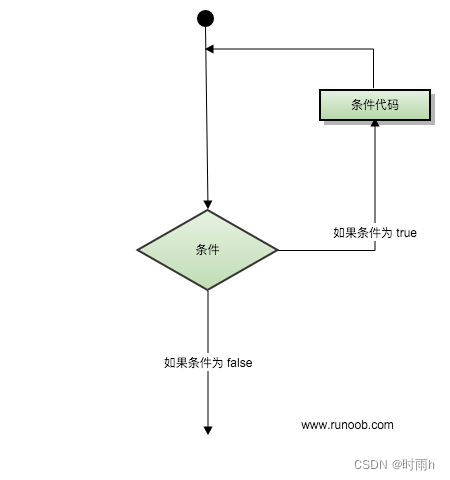### 无限循环

``````如果条件永远不为假，则循环将变成无限循环。for 循环在传统意义上可用于实现无限循环。由于构成循环的三个表达式中任何一个都不是必需的，您可以将某些条件表达式留空来构成一个无限循环。实例
#include <iostream>
using namespace std;int main ()
{for( ; ; ){printf("This loop will run forever.\n");}return 0;
}
``````

## C++ 判断

``````#include<iostream>
using namespace std;int main(){int a,b,c,d,max;cout<<"请输入三个数字：";cin>>a>>b>>c;max=(d=a>=b?a:b)>=c?d:c;cout<<"最大值为："<<max<<endl;return 0;
}
``````

``````#include<iostream>
#include <iomanip>
using namespace std;int main()
{int a, b, c, d, m, n,z;a = 10;b = 20;c = 30;d = 40;m = a > b ? a : b;n = c > d ? c : d;z = m > n ? m : n;cout<<"最大值为："<<z<<endl;return 0;
}
``````

``````#include<iostream>
using namespace std;int main(){int a,max;cout<<"请输入四个数字：";cin>>a>>a>>a>>a;max = a[a[a>a?1:2]>a[a>a?3:4]?a>a?1:2:a>a?3:4];cout<<"最大值为："<<max<<endl;return 0;
}
``````

## C++ 函数

C++ 函数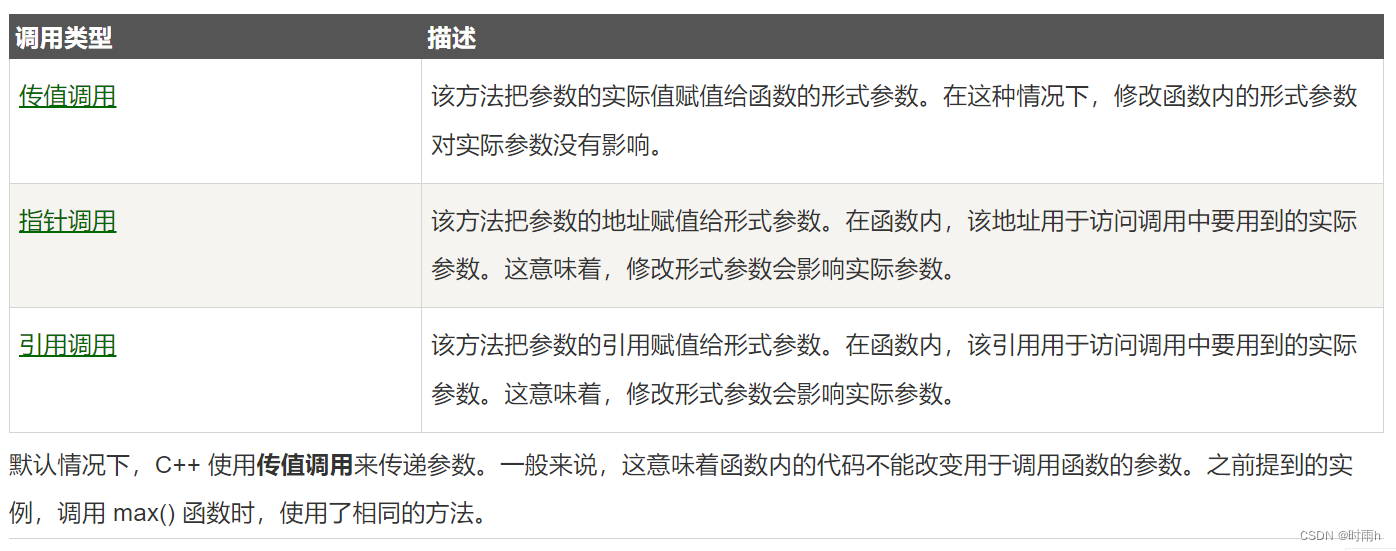``````#include <iostream>
using namespace std;// 函数声明
void swap(int x, int y);int main ()
{// 局部变量声明int a = 100;int b = 200;cout << "交换前，a 的值：" << a << endl;cout << "交换前，b 的值：" << b << endl;// 调用函数来交换值swap(a, b);cout << "交换后，a 的值：" << a << endl;cout << "交换后，b 的值：" << b << endl;return 0;
}
``````

``````交换前，a 的值： 100

``````

### C++ 指针调用

``````#include <iostream>
using namespace std;// 函数声明
void swap(int *x, int *y);int main ()
{// 局部变量声明int a = 100;int b = 200;cout << "交换前，a 的值：" << a << endl;cout << "交换前，b 的值：" << b << endl;/* 调用函数来交换值* &a 表示指向 a 的指针，即变量 a 的地址 * &b 表示指向 b 的指针，即变量 b 的地址 */swap(&a, &b);cout << "交换后，a 的值：" << a << endl;cout << "交换后，b 的值：" << b << endl;return 0;
}
``````

``````交换前，a 的值： 100

``````

### 参数的默认值

``````#include <iostream>
using namespace std;int sum(int a, int b=20)
{int result;result = a + b;return (result);
}int main ()
{// 局部变量声明int a = 100;int b = 200;int result;// 调用函数来添加值result = sum(a, b);cout << "Total value is :" << result << endl;// 再次调用函数result = sum(a);cout << "Total value is :" << result << endl;return 0;
}
``````

``````Total value is :300
Total value is :120
``````

## C++ 数学运算

C++ 内置了丰富的数学函数，可对各种数字进行运算。下表列出了 C++ 中一些有用的内置的数学函数。

1 double cos(double);

2 double sin(double);

3 double tan(double);

4 double log(double);

5 double pow(double, double);

6 double hypot(double, double);

7 double sqrt(double);

8 int abs(int);

9 double fabs(double);

10 double floor(double);

#include
#include
using namespace std;

int main ()
{
// 数字定义
short s = 10;
int i = -1000;
long l = 100000;
float f = 230.47;
double d = 200.374;

// 数学运算
cout << “sin(d) :” << sin(d) << endl;
cout << “abs(i) :” << abs(i) << endl;
cout << “floor(d) :” << floor(d) << endl;
cout << “sqrt(f) :” << sqrt(f) << endl;
cout << “pow( d, 2) :” << pow(d, 2) << endl;

return 0;
}

sin(d) :-0.634939
abs(i) :1000
floor(d) :200
sqrt(f) :15.1812
pow( d, 2 ) :40149.7

## C++ 随机数

#include
#include
#include

using namespace std;

int main ()
{
int i,j;

// 设置种子
srand( (unsigned)time( NULL ) );

/* 生成 10 个随机数 */
for( i = 0; i < 10; i++ )
{
// 生成实际的随机数
j= rand();
cout <<"随机数： " << j << endl;
}

return 0;
}

## C++ 数组

C++ 支持数组数据结构，它可以存储一个固定大小的相同类型元素的顺序集合。数组是用来存储一系列数据，但它往往被认为是一系列相同类型的变量。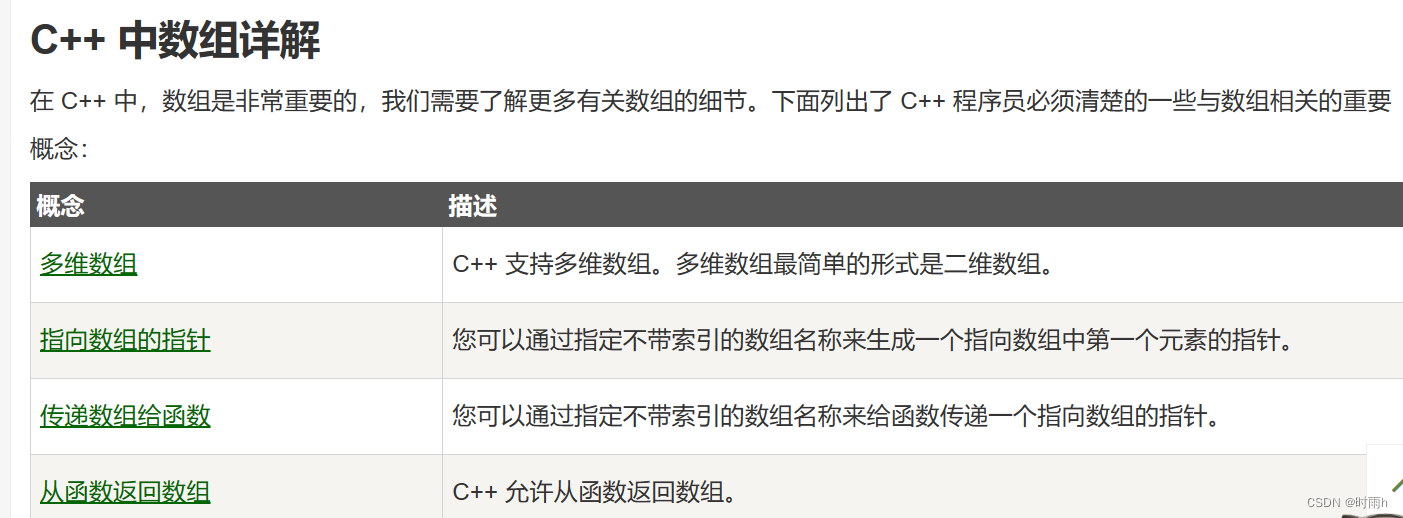## C++ 字符串

C++ 提供了以下两种类型的字符串表示形式：

C 风格字符串
C++ 引入的 string 类类型

C++ 字符串

### C++ 中的 String 类

C++ 标准库提供了 string 类类型，支持上述所有的操作，另外还增加了其他更多的功能。我们将学习 C++ 标准库中的这个类，现在让我们先来看看下面这个实例：

``````#include <iostream>
#include <string>using namespace std;int main ()
{string str1 = "runoob";string str2 = "google";string str3;int  len ;// 复制 str1 到 str3str3 = str1;cout << "str3 : " << str3 << endl;// 连接 str1 和 str2str3 = str1 + str2;cout << "str1 + str2 : " << str3 << endl;// 连接后，str3 的总长度len = str3.size();cout << "str3.size() :  " << len << endl;return 0;
}
``````

``````str3 : runoob
str3.size() :  12``````

string类提供了一系列针对字符串的操作，比如：

1. append() – 在字符串的末尾添加字符
2. find() – 在字符串中查找字符串
3. insert() – 插入字符
4. length() – 返回字符串的长度
5. replace() – 替换字符串
6. substr() – 返回某个子字符串

7. 下面是关于string类的实例：
``````#include <iostream>
#include <string>
using namespace std;int main()
{//定义一个string类对象string http = "www.runoob.com";//打印字符串长度cout<<http.length()<<endl;//拼接http.append("/C++");cout<<http<<endl; //打印结果为：www.runoob.com/C++//删除int pos = http.find("/C++"); //查找"C++"在字符串中的位置cout<<pos<<endl;http.replace(pos, 4, "");   //从位置pos开始，之后的4个字符替换为空，即删除cout<<http<<endl;//找子串runoobint first = http.find_first_of("."); //从头开始寻找字符'.'的位置int last = http.find_last_of(".");   //从尾开始寻找字符'.'的位置cout<<http.substr(first+1, last-first-1)<<endl; //提取"runoob"子串并打印return 0;
}
``````

## C++ 指针

``````#include <iostream>using namespace std;int main ()
{int  var1;char var2;cout << "var1 变量的地址： ";cout << &var1 << endl;cout << "var2 变量的地址： ";cout << &var2 << endl;return 0;
}
``````

var1 变量的地址： 0xbfebd5c0
var2 变量的地址： 0xbfebd5b6

### 什么是指针？

type *var-name;

int ip; / 一个整型的指针 */
double dp; / 一个 double 型的指针 */
float fp; / 一个浮点型的指针 */
char ch; / 一个字符型的指针 */

### C++ 中使用指针

``````#include <iostream>using namespace std;int main ()
{int  var = 20;   // 实际变量的声明int  *ip;        // 指针变量的声明ip = &var;       // 在指针变量中存储 var 的地址cout << "Value of var variable: ";cout << var << endl;// 输出在指针变量中存储的地址cout << "Address stored in ip variable: ";cout << ip << endl;// 访问指针中地址的值cout << "Value of *ip variable: ";cout << *ip << endl;return 0;
}

Address stored in ip variable: 0xbfc601ac
Value of *ip variable: 20
``````## C++ 引用

### C++ 引用 vs 指针

C++ 中创建引用

int i = 17;

int& r = i;
double& s = d;

``````#include <iostream>using namespace std;int main ()
{// 声明简单的变量int    i;double d;// 声明引用变量int&    r = i;double& s = d;i = 5;cout << "Value of i : " << i << endl;cout << "Value of i reference : " << r  << endl;d = 11.7;cout << "Value of d : " << d << endl;cout << "Value of d reference : " << s  << endl;return 0;
}
``````

``````Value of i : 5
Value of i reference : 5
Value of d : 11.7
Value of d reference : 11.7
``````
``````int& r = i; 和 int r = i; 不同之处应该是内存的分配吧，后者会再开辟一个内存空间#include <iostream>using namespace std;int main ()
{int i;int& r = i;i = 5;cout << "Value of i : " << i << endl;cout << "Value of i reference : " << r  << endl;cout << "Addr of i: " << &i << endl;cout << "Addr of r: " << &r << endl;int x;int y = x;x = 6;cout << "Value of x : " << x << endl;cout << "Value of y : " << y  << endl;cout << "Addr of x: " << &x << endl;cout << "Addr of y: " << &y << endl;return 0;
}

Value of i reference : 5
Value of x : 6
Value of y : 32767
``````

## 当前日期和时间

``````#include <iostream>
#include <ctime>using namespace std;int main( )
{// 基于当前系统的当前日期/时间time_t now = time(0);// 把 now 转换为字符串形式char* dt = ctime(&now);cout << "本地日期和时间：" << dt << endl;// 把 now 转换为 tm 结构tm *gmtm = gmtime(&now);dt = asctime(gmtm);cout << "UTC 日期和时间："<< dt << endl;
}
``````

``````本地日期和时间：Sat Jan  8 20:07:41 2011UTC 日期和时间：Sun Jan  9 03:07:41 2011
``````

## 使用结构 tm 格式化时间

tm 结构在 C/C++ 中处理日期和时间相关的操作时，显得尤为重要。tm 结构以 C 结构的形式保存日期和时间。大多数与时间相关的函数都使用了 tm 结构。下面的实例使用了 tm 结构和各种与日期和时间相关的函数。

``````#include <iostream>
#include <ctime>using namespace std;int main( )
{// 基于当前系统的当前日期/时间time_t now = time(0);cout << "1970 到目前经过秒数:" << now << endl;tm *ltm = localtime(&now);// 输出 tm 结构的各个组成部分cout << "年: "<< 1900 + ltm->tm_year << endl;cout << "月: "<< 1 + ltm->tm_mon<< endl;cout << "日: "<<  ltm->tm_mday << endl;cout << "时间: "<< ltm->tm_hour << ":";cout << ltm->tm_min << ":";cout << ltm->tm_sec << endl;
}
``````

``````1970 到目前时间:1503564157

``````

## C++ 基本的输入输出

C++ 标准库提供了一组丰富的输入/输出功能，我们将在后续的章节进行介绍。本章将讨论 C++ 编程中最基本和最常见的 I/O 操作。

C++ 的 I/O 发生在流中，流是字节序列。如果字节流是从设备（如键盘、磁盘驱动器、网络连接等）流向内存，这叫做输入操作。如果字节流是从内存流向设备（如显示屏、打印机、磁盘驱动器、网络连接等），这叫做输出操作。

I/O 库头文件

### 头文件 函数和描述

#include

using namespace std;

int main( )
{
char str[] = “Hello C++”;

cout << "Value of str is : " << str << endl;
}

Value of str is : Hello C++
C++ 编译器根据要输出变量的数据类型，选择合适的流插入运算符来显示值。<< 运算符被重载来输出内置类型（整型、浮点型、double 型、字符串和指针）的数据项。

### 标准输入流（cin）

#include

using namespace std;

int main( )
{
char name;

cout << "请输入您的名称： ";
cin >> name;
cout << "您的名称是： " << name << endl;

}

C++ 编译器根据要输入值的数据类型，选择合适的流提取运算符来提取值，并把它存储在给定的变量中。

cin >> name >> age;

cin >> name;
cin >> age;

cerr 也是与流插入运算符 << 结合使用的，如下所示：

#include

using namespace std;

int main( )
{
char str[] = “Unable to read…”;

cerr << "Error message : " << str << endl;
}

Error message : Unable to read…

clog 也是与流插入运算符 << 结合使用的，如下所示：

#include

using namespace std;

int main( )
{
char str[] = “Unable to read…”;

clog << "Error message : " << str << endl;
}

Error message : Unable to read…

## C++ 数据结构

C/C++ 数组允许定义可存储相同类型数据项的变量，但是结构是 C++ 中另一种用户自定义的可用的数据类型，它允许您存储不同类型的数据项。

Title ：标题
Author ：作者
Subject ：类目
Book ID ：书的 ID

struct type_name {
member_type1 member_name1;
member_type2 member_name2;
member_type3 member_name3;
.
.
} object_names;
type_name 是结构体类型的名称，member_type1 member_name1 是标准的变量定义，比如 int i; 或者 float f; 或者其他有效的变量定义。在结构定义的末尾，最后一个分号之前，您可以指定一个或多个结构变量，这是可选的。下面是声明一个结构体类型 Books，变量为 book：

struct Books
{
char title;
char author;
char subject;
int book_id;
} book;

#include
#include

using namespace std;

// 声明一个结构体类型 Books
struct Books
{
char title;
char author;
char subject;
int book_id;
};

int main( )
{
Books Book1; // 定义结构体类型 Books 的变量 Book1
Books Book2; // 定义结构体类型 Books 的变量 Book2

// Book1 详述
strcpy( Book1.title, “C++ 教程”);
strcpy( Book1.author, “Runoob”);
strcpy( Book1.subject, “编程语言”);
Book1.book_id = 12345;

// Book2 详述
strcpy( Book2.title, “CSS 教程”);
strcpy( Book2.author, “Runoob”);
strcpy( Book2.subject, “前端技术”);
Book2.book_id = 12346;

// 输出 Book1 信息
cout << "第一本书标题 : " << Book1.title <<endl;
cout << "第一本书作者 : " << Book1.author <<endl;
cout << "第一本书类目 : " << Book1.subject <<endl;
cout << "第一本书 ID : " << Book1.book_id <<endl;

// 输出 Book2 信息
cout << "第二本书标题 : " << Book2.title <<endl;
cout << "第二本书作者 : " << Book2.author <<endl;
cout << "第二本书类目 : " << Book2.subject <<endl;
cout << "第二本书 ID : " << Book2.book_id <<endl;

return 0;
}

#include
#include

using namespace std;
void printBook( struct Books book );

// 声明一个结构体类型 Books
struct Books
{
char title;
char author;
char subject;
int book_id;
};

int main( )
{
Books Book1; // 定义结构体类型 Books 的变量 Book1
Books Book2; // 定义结构体类型 Books 的变量 Book2

``````// Book1 详述
``````

strcpy( Book1.title, “C++ 教程”);
strcpy( Book1.author, “Runoob”);
strcpy( Book1.subject, “编程语言”);
Book1.book_id = 12345;

// Book2 详述
strcpy( Book2.title, “CSS 教程”);
strcpy( Book2.author, “Runoob”);
strcpy( Book2.subject, “前端技术”);
Book2.book_id = 12346;

// 输出 Book1 信息
printBook( Book1 );

// 输出 Book2 信息
printBook( Book2 );

return 0;
}
void printBook( struct Books book )
{
cout << "书标题 : " << book.title <<endl;
cout << "书作者 : " << book.author <<endl;
cout << "书类目 : " << book.subject <<endl;
cout << "书 ID : " << book.book_id <<endl;
}

struct Books *struct_pointer;

struct_pointer = &Book1;

struct_pointer->title;

#include
#include

using namespace std;
void printBook( struct Books *book );

struct Books
{
char title;
char author;
char subject;
int book_id;
};

int main( )
{
Books Book1; // 定义结构体类型 Books 的变量 Book1
Books Book2; // 定义结构体类型 Books 的变量 Book2

``````// Book1 详述
``````

strcpy( Book1.title, “C++ 教程”);
strcpy( Book1.author, “Runoob”);
strcpy( Book1.subject, “编程语言”);
Book1.book_id = 12345;

// Book2 详述
strcpy( Book2.title, “CSS 教程”);
strcpy( Book2.author, “Runoob”);
strcpy( Book2.subject, “前端技术”);
Book2.book_id = 12346;

// 通过传 Book1 的地址来输出 Book1 信息
printBook( &Book1 );

// 通过传 Book2 的地址来输出 Book2 信息
printBook( &Book2 );

return 0;
}
// 该函数以结构指针作为参数
void printBook( struct Books *book )
{
cout << "书标题 : " << book->title <<endl;
cout << "书作者 : " << book->author <<endl;
cout << "书类目 : " << book->subject <<endl;
cout << "书 ID : " << book->book_id <<endl;
}

typedef 关键字

typedef struct Books
{
char title;
char author;
char subject;
int book_id;
}Books;

Books Book1, Book2;

typedef long int *pint32;

pint32 x, y, z;
x, y 和 z 都是指向长整型 long int 的指针。